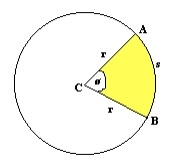# Area of a Circular Sector?

CServer Side ProgrammingProgramming

#### C in Depth: The Complete C Programming Guide for Beginners

45 Lectures 4.5 hours

#### Practical C++: Learn C++ Basics Step by Step

Most Popular

50 Lectures 4.5 hours

#### Master C and Embedded C Programming- Learn as you go

66 Lectures 5.5 hours

A circular sector also known as circle sector / sector of a circle is the portion of the circle that is inscribed by between 2 radii. This area is enclosed between two radii and an arc. To find the area inscribed we need to find the angle that is between the two radii. The total area is equal to 360o of angle. To find the area for an angle we will multiply the area by θ/360. This given the area of section inscrible.

Where θ is the angle between the two radii in degree.Area of a sector of a circle = π*r*r*(θ/360).

## Example

Area of a sector of a circle of radius = 5 with angle of 60o is 13.083

Area = (3.14*5*5)*(60/360) = 13.03

## Example Code

Live Demo

#include <stdio.h>
int main(void) {
int r = 5;
int angle = 60;
float pie = 3.14;
float area = (float)(pie*r*r*angle/360);
printf("The area of sector of a circle of radius %d with an angle of %d is %f", r,angle,area);
return 0;
}

## Output

The area of sector of a circle of radius 5 with an angle of 60 is 13.083333